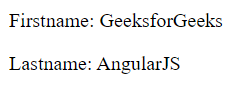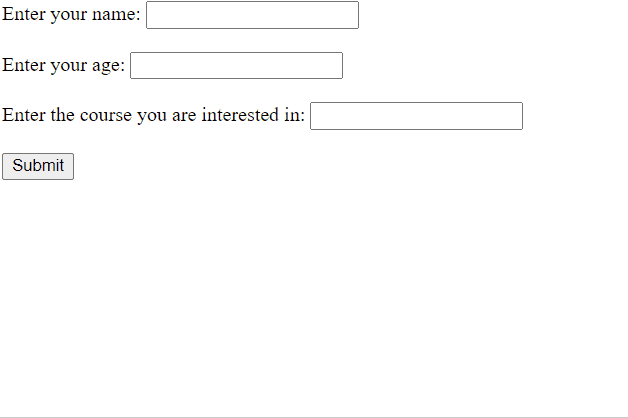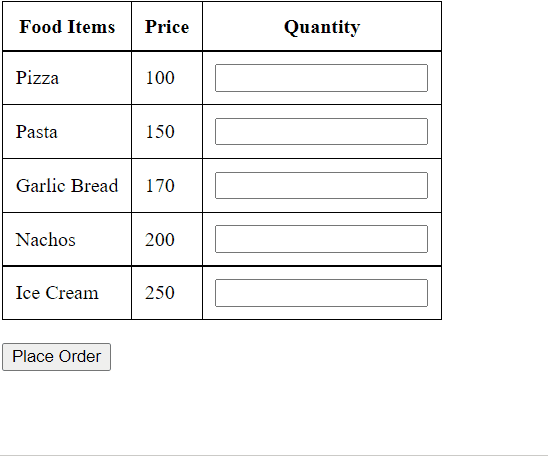GFG App
Open AppBrowser
Continue

# How many types of data bindings in AngularJS ?

In this article, we will know about the concept of data binding, along with different types of binding available in AngularJS.

The Data Binding refers to the synchronization of data between the model and view. Synchronizing data is imperative for keeping the data being displayed to the user and the data being stored updated at all times. In AngularJS, Data Binding is an important concept as it acts as a bridge between the view and the logic of the AngularJS app. Data Binding In AngularJS is achieved by using Directives.

There are 2 major components of Data Binding in AngularJS:

• Model: It is responsible for maintaining data in the application.
• View: It is the HTML container where the app is displayed to the user.

AngularJS provides two types of Data Binding:

• One-way data binding
• Two-way data binding

We will discuss both of them in detail with examples.

One Way Data Binding: In one-way data binding, the flow of data is in one direction only i.e. from model to view. A value is taken from the data model, inserted in an HTML element, and displayed to the user. But there is no way to update the model according to the input given by the user which means that the data can’t flow from the view to the model.

One-Way Data Binding can be achieved by:

• Interpolation
• Using ng-bind directive

Interpolation: Interpolation is a one-way data-binding technique that is used to transfer the data from a TypeScript code to an HTML template (view). It uses the template expression in double curly braces to display the data from the component to the view.

Syntax:

`{{expression}}`

Example: This example describes the One-way data binding using double braces expressions.

## HTML

 ` ` `<``html``> ` ` `  `<``head``> ` `    ``<``title``>Data Binding Example ` `    ``<``script` `src``= ` `"https://ajax.googleapis.com/ajax/libs/angularjs/1.6.9/angular.min.js"``> ` `    `` ` ` ` ` `  `<``body` `ng-app``=``"myApp"``> ` `    ``<``div` `ng-controller``=``"myCtrl"``> ` `        ``<``h1``>{{title1}} ` `        ``<``h2``>{{title2}} ` `        ``<``p``>{{description}} ` `    `` ` `    ``<``script` `type``=``"text/javascript"``> ` `        ``var app = angular.module("myApp", []); ` `        ``app.controller("myCtrl", function(\$scope) { ` `            ``\$scope.title1 = "GeeksforGeeks"; ` `            ``\$scope.title2 = "AngularJs Data Binding"; ` `            ``\$scope.description ="Data Binding refers " ` `              ``+ "to the synchronization of data " ` `              ``+ "between the model and view"; ` `        ``}); ` `    `` ` ` ` ``

Explanation: In the above example, we have used expressions to bind the data from the model to the view. In the <script> tag we have created a module named “myApp”. To this module, we have added a controller “myCtrl”. We have added properties to this controller like title1, title2, and description. Then we have specified these properties in the HTML elements where we want to show the data to the users.

Output:

Using ng-bind directive: The ng-bind Directive in AngularJS is used to bind/replace the text content of any particular HTML element with the value that is entered in the given expression. The value of specified HTML content updates whenever the value of the expression changes in ng-bind directive.

Syntax:

`<element ng-bind="expression"> Contents... </element>`

Example: This example describe the One-way data binding using ng-bind directive.

## HTML

 ` ` `<``html``> ` ` `  `<``head``> ` `    ``<``title``>Data Binding Example ` `    ``<``script` `src``= ` `"https://ajax.googleapis.com/ajax/libs/angularjs/1.6.9/angular.min.js"``> ` `    `` ` ` ` ` `  `<``body` `ng-app``=``"myApp"``> ` `    ``<``div` `ng-controller``=``"myCtrl"``> ` `        ``<``p``>Firstname:  ` `            ``<``span` `ng-bind``=``"firstname"``> ` `        `` ` ` `  `        ``<``p``>Lastname:  ` `            ``<``span` `ng-bind``=``"lastname"``> ` `        `` ` `    `` ` `    ``<``script` `type``=``"text/javascript"``> ` `        ``var app = angular.module("myApp", []); ` `        ``app.controller("myCtrl", function(\$scope) { ` `            ``\$scope.firstname = "GeeksforGeeks"; ` `            ``\$scope.lastname = "AngularJS"; ` `        ``}); ` `    `` ` ` ` ``

Explanation: In the above example, we have used ng-bind to bind data from the model to the view. In the <script> tag we have added properties to the controller like firstname and lastname. We have specified the ng-bind directive in the HTML tags where we want to display the data. In the ng-bind directive, we have specified the property we want to display.

Output:Data Binding using the ng-bind directive

Two Way Data Binding: In this type of data binding, the flow of data is bidirectional i.e the data can flow from the model to the view as well as from the view to the model. In simple words, we can say that when the data in the model changes, the changes are reflected in the view and when the data in the view changes the model is also updated. The view and model are updated at all times.

Two-way data binding is achieved by using the ng-model directive. The ng-model directive transfers data from the controller to the view and vice versa.

Example: In this example, we have created a form asking for the user’s name, age, and the course they are interested in. After the user gives the input for all the fields and clicks on the submit button the details entered by the user are displayed.

## HTML

 ` ` `<``html``> ` ` `  `<``head``> ` `    ``<``title``>Data Binding Example ` `    ``<``script` `src``= ` `"https://ajax.googleapis.com/ajax/libs/angularjs/1.6.9/angular.min.js"``> ` `    `` ` ` ` ` `  `<``body` `ng-app``=``"myApp"``> ` `    ``<``div` `ng-controller``=``"myCtrl"``> ` `        ``<``form``> ` `            ``<``label``>Enter your name: ` `            ``<``input` `type``=``"text"` `ng-model``=``"name"``> ` `            ``<``br``> ` `            ``<``label``>Enter your age: ` `            ``<``input` `type``=``"number"` `ng-model``=``"age"``> ` `            ``<``br``> ` `            ``<``label``>Enter the course you are interested in: ` `            ``<``input` `type``=``"text"` `ng-model``=``"course"``> ` `            ``<``br``> ` `            ``<``input` `type``=``"submit"` `ng-click``=``"details()"``>  ` `        `` ` `        ``<``div` `ng-show``=``"showdetails"``> ` `            ``<``h1``>Details entered by the user: ` `            ``<``p``>Name: {{name}} ` `            ``<``p``>Age: {{age}} ` `            ``<``p``>Course: {{course}} ` `        `` ` `    `` ` `    ``<``script` `type``=``"text/javascript"``> ` `        ``var app = angular.module("myApp", []); ` `        ``app.controller("myCtrl", function(\$scope) { ` `            ``\$scope.showdetails = false; ` `            ``\$scope.details = function() { ` `                ``\$scope.showdetails = true; ` `            ``} ` `        ``}); ` `    `` ` ` ` ``

Explanation: We have specified the ng-model directive in all the input fields so as to create a model property for each of them. Initially in the controller, the value for the property “details” is false. After the submit button is clicked, the function details() is called which changes the value of the property “details” to true. In the <div> tag we have specified the ng-show directive which shows the <div> tag if the value of “details” is true. So as the user enters the details and clicks on the submit button the details are displayed using expressions.

Output:Two Way Data Binding Example

Example: In this example, the user is supposed to enter the quantity for each product they want to order, and accordingly the price will be calculated and displayed to the user.

## HTML

 ` ` `<``html``> ` ` `  `<``head``> ` `    ``<``title``>>Data Binding Example ` `    ``<``script` `src``= ` `"https://ajax.googleapis.com/ajax/libs/angularjs/1.6.9/angular.min.js"``> ` `    `` ` `    ``<``style``> ` `        ``table { ` `            ``border: 1px solid black; ` `            ``border-collapse: collapse; ` `        ``} ` `         `  `        ``tr,    th,    td { ` `            ``border: 1px solid black; ` `            ``border-collapse: collapse; ` `            ``padding: 10px; ` `        ``} ` `    `` ` ` ` ` `  `<``body` `ng-app``=``"myApp"``> ` `    ``<``div` `ng-controller``=``"myCtrl"``> ` `        ``<``table``> ` `            ``<``tr``> ` `                ``<``th``>Food Items ` `                ``<``th``>Price ` `                ``<``th``>Quantity ` `            `` ` `            ``<``tr``> ` `                ``<``td``>Pizza ` `                ``<``td``>100 ` `                ``<``td``> ` `                    ``<``input` `type``=``"number"`  `                           ``ng-model``=``"pizza"``> ` `                `` ` `            `` ` `            ``<``tr``> ` `                ``<``td``>Pasta ` `                ``<``td``>150 ` `                ``<``td``> ` `                    ``<``input` `type``=``"number"`  `                           ``ng-model``=``"pasta"``> ` `                `` ` `            `` ` `            ``<``tr``> ` `                ``<``td``>Garlic Bread ` `                ``<``td``>170 ` `                ``<``td``> ` `                    ``<``input` `type``=``"number"`  `                           ``ng-model``=``"garlicbread"``> ` `                `` ` `            `` ` `            ``<``tr``> ` `                ``<``td``>Nachos ` `                ``<``td``>200 ` `                ``<``td``> ` `                    ``<``input` `type``=``"number"`  `                           ``ng-model``=``"nachos"``> ` `                `` ` `            `` ` `            ``<``tr``> ` `                ``<``td``>Ice Cream ` `                ``<``td``>250 ` `                ``<``td``> ` `                    ``<``input` `type``=``"number"`  `                           ``ng-model``=``"icecream"``> ` `                `` ` `            `` ` `        `` ` `        ``<``br``> ` `        ``<``button` `ng-click``=``"calculate()"``> ` `            ``Place Order ` `        `` ` `        ``<``div` `ng-show``=``"amt!=0"``> ` `            ``<``h1``> ` `                ``Total amount to be paid: {{amt}} ` `            ``  ` `        `` ` `    `` ` `    ``<``script` `type``=``"text/javascript"``> ` `        ``var app = angular.module("myApp", []); ` `        ``app.controller("myCtrl", function(\$scope) { ` `            ``\$scope.title = "Food ordering"; ` `            ``\$scope.amt = 0; ` `            ``\$scope.calculate = function() { ` `                ``\$scope.amt = 100 * \$scope.pizza  ` `                           ``+ 150 * \$scope.pasta  ` `                           ``+ 170 * \$scope.garlicbread  ` `                           ``+ 200 * \$scope.nachos  ` `                           ``+ 250 * \$scope.icecream; ` `            ``} ` `        ``}); ` `    `` ` ` ` ``

Explanation: In the above example, we have used data binding in order to take data from the user from the view, pass it to the controller, the controller performs the processing using the model properties and then passes it to the view for the data to be shown to the users.

Output:My Personal Notes arrow_drop_up